# T3 - page 30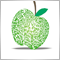106

Yes ,just to add 2 x ATR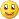216615

sohocool:
Hi Mladen,

Do you think is it possible to do Adaptive Hull Moving Average V2 ???

Decimal period seems to work .Regards

Hull is based on LWMA and since LWMA is strictly bars based it would not be so smooth in changes as EMA based ones, for example (sub-periods of the 3 LWMAs calculated to get HULL must be integer values) . If we replace LWMA in hull with EMA it might be worth a shot (and if we avoid rounding of sub-periods calculated). True that it would not be a HULL then any more, but maybe it would be a good one, and then it would be perfect for adapting106

mladen:
Hull is based on LWMA and since LWMA is strictly bars based it would not be so smooth in changes as EMA based ones, for example (sub-periods of the 3 LWMAs calculated to get HULL must be integer values) . If we replace LWMA in hull with EMA it might be worth a shot (and if we avoid rounding of sub-periods calculated). True that it would not be a HULL then any more, but maybe it would be a good one, and then it would be perfect for adapting

Thanks ,i will try to do with Ema .216615

sohocool:
Thanks ,i will try to do with Ema .Just don't use the built in iMA() for that since it rounds the periods to integer values

Use this function (or something similar) :

//------------------------------------------------------------------

//

//------------------------------------------------------------------

//

//

//

//

//

double workEma[];

double iEma(double price, double period, int r, int instanceNo=0)

{

if (ArrayRange(workEma,0)!= Bars) ArrayResize(workEma,Bars); r = Bars-r-1;

//

//

//

//

//

double alpha = 2.0 / (1.0+period);

workEma[r] = workEma[r-1]+alpha*(price-workEma[r-1]);

return(workEma[r]);

}

in 3 different instances of calculation. Parameters are simple : price, period, index and instance number106

mladen:
Just don't use the built in iMA() for that since it rounds the periods to integer values

Use this function (or something similar) :

//------------------------------------------------------------------

//

//------------------------------------------------------------------

//

//

//

//

//

double workEma[];

double iEma(double price, double period, int r, int instanceNo=0)

{

if (ArrayRange(workEma,0)!= Bars) ArrayResize(workEma,Bars); r = Bars-r-1;

//

//

//

//

//

double alpha = 2.0 / (1.0+period);

workEma[r] = workEma[r-1]+alpha*(price-workEma[r-1]);

return(workEma[r]);

}

in 3 different instances of calculation. Parameters are simple : price, period, index and instance number

I must delete and paste here ???

double iT3(double price, double period, double hot, bool clean, int i, int instanceNo=0)

{

if (ArrayRange(workT3,0) !=Bars) ArrayResize(workT3,Bars);

if (ArrayRange(workT3Coeffs,0) < (instanceNo+1)) ArrayResize(workT3Coeffs,instanceNo+1);

if (workT3Coeffs[_period] != period)

{

workT3Coeffs[_period] = period;

double a = hot;

workT3Coeffs[_c1] = -a*a*a;

workT3Coeffs[_c2] = 3*a*a+3*a*a*a;

workT3Coeffs[_c3] = -6*a*a-3*a-3*a*a*a;

workT3Coeffs[_c4] = 1+3*a+a*a*a+3*a*a;

if (clean)

workT3Coeffs[_alpha] = 2.0/(2.0 + (period-1.0)/2.0);

else workT3Coeffs[_alpha] = (MathCos(2*Pi/period)+MathSin(2*Pi/period)-1)/MathCos(2*Pi/period);

}216615

sohocool:
I must delete and paste here ???

double iT3(double price, double period, double hot, bool clean, int i, int instanceNo=0)

{

if (ArrayRange(workT3,0) !=Bars) ArrayResize(workT3,Bars);

if (ArrayRange(workT3Coeffs,0) < (instanceNo+1)) ArrayResize(workT3Coeffs,instanceNo+1);

if (workT3Coeffs[_period] != period)

{

workT3Coeffs[_period] = period;

double a = hot;

workT3Coeffs[_c1] = -a*a*a;

workT3Coeffs[_c2] = 3*a*a+3*a*a*a;

workT3Coeffs[_c3] = -6*a*a-3*a-3*a*a*a;

workT3Coeffs[_c4] = 1+3*a+a*a*a+3*a*a;

if (clean)

workT3Coeffs[_alpha] = 2.0/(2.0 + (period-1.0)/2.0);

else workT3Coeffs[_alpha] = (MathCos(2*Pi/period)+MathSin(2*Pi/period)-1)/MathCos(2*Pi/period);

}

sohocool

Posted one version how it can be done (that is still not an adaptive version) here : https://www.mql5.com/en/forum/174961/page10

Now you have to make it adaptive (after all it was your idea)106

sohocool:
Hi Mladen,

The adaptive function is very interesting.

I have do the adaptive T3 with "Swiss Army " Alpha .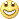We can choose 2 alphas : clean or Swiss army.

Regards.

PS: If you want normal alpha period ,you can use clean alpha : 2 x period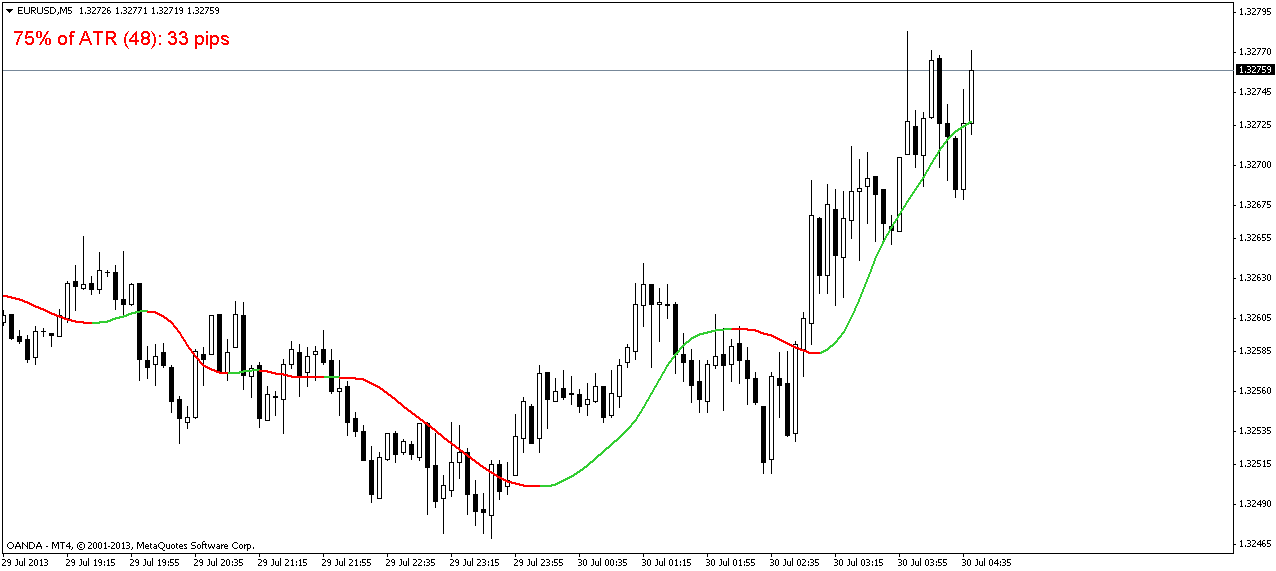Hi all,

i have just add ATR channel .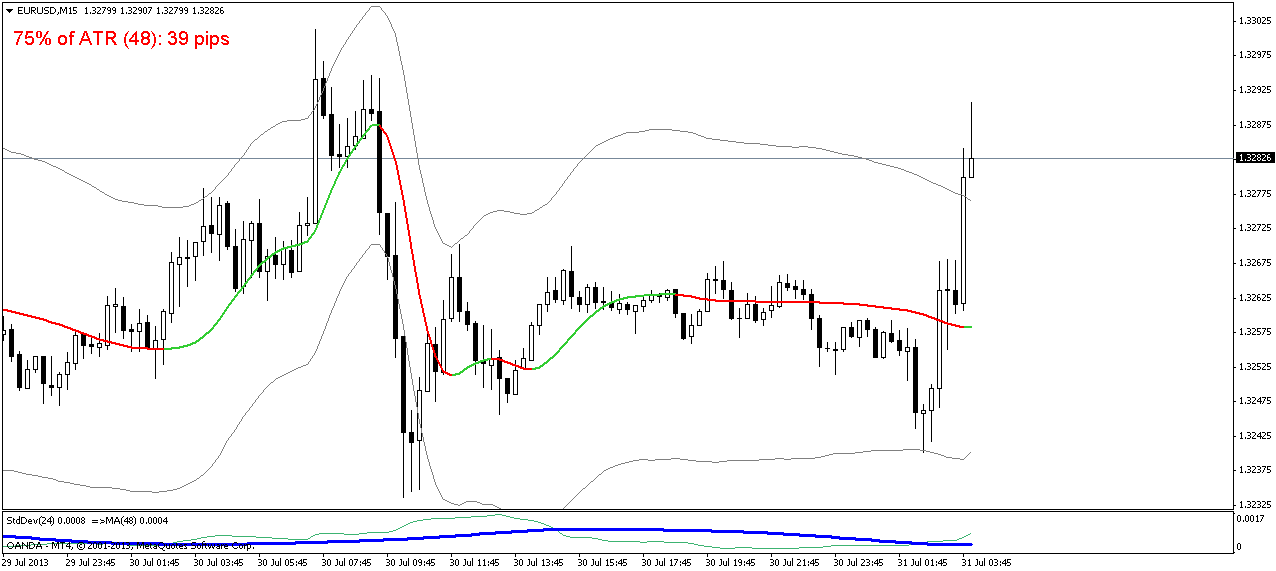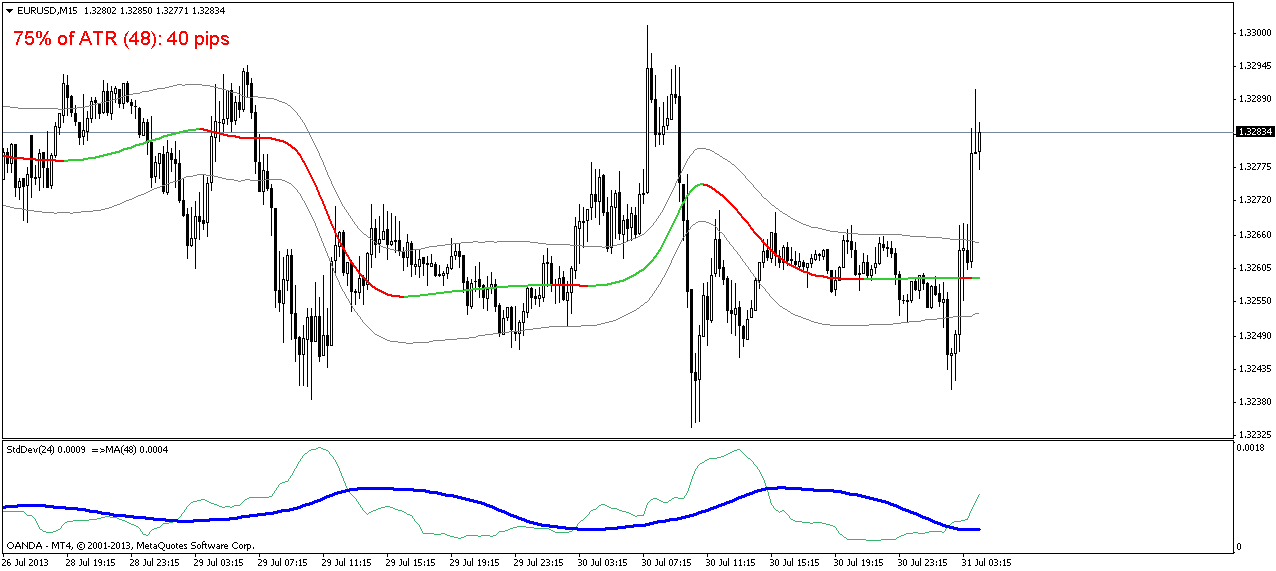Files:898

mladen:
T3 indicator using standard deviation for adapting (T3 is very good for adapting, since its calculating length does not need to be an integer - for example you can calculate a nnn.5 T3 - and that gives a perfectly smooth T3 value even when adapting is applied to it which is not a case with some other averages types adapting)

PS: attaching the ex4 too for those that might have problems compiling the source. Even though the indicator is written from the first letter to the last by me, my build was refusing to compile it for some time. Now, out of the blue, it compiles it with no problem, so I am not sure that someone else will not have the same problem I had. For that case download the the ex4 (it is built with build 500)

PS: when comparing to "regular" T3 indicators set the T3Original to false (since most of the T3 indicators use Fulks/Matulich calculation and not the orginal Tim Tillson calculation)

Dear Mladen

Would it be possible for you to convert attached indicator with 'Adaptive T3 calculations'!

Thanks for any help

secretcode

Files:
ma_i-ca.mq4  2 kb216615

secretcode:
Dear Mladen

Would it be possible for you to convert attached indicator with 'Adaptive T3 calculations'!

Thanks for any help

secretcode

secretcode

Here you goUse d same default parameters as the original indicator (so that they can easily be compared - as expected this one is much faster)

Files:
t3_mai_ca.gif  36 kb57

Hi Mladen,

Have you ever thought of making a library file collecting your beautiful coding methods of smoothing price, or adapting to market cycles, etc. ? I think such file would be very useful in many ways, at least make coding structure in many of your indicators cleaner and clearer (they are very clean and clear already, i knowbut always wish for better and search for more excellent).

I personally love to have such a lib file from you, the same as your famous DynamicZone.dll . They all make coding world more and more interesting and convenient, to me at least.